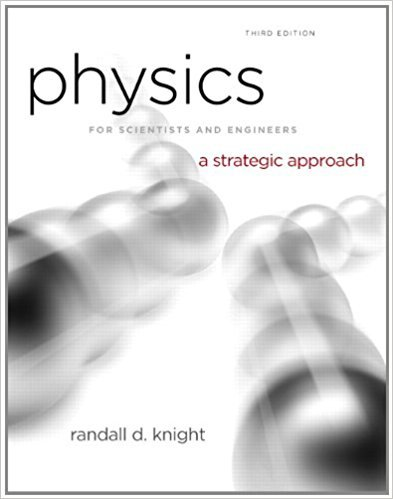×
Get Full Access to Physics For Scientists And Engineers: A Strategic Approach With Modern Physics - 3 Edition - Chapter 6 - Problem 61p
Get Full Access to Physics For Scientists And Engineers: A Strategic Approach With Modern Physics - 3 Edition - Chapter 6 - Problem 61p

×

# Astronauts in space “weigh” themselves by oscillating on aISBN: 9780321740908 69

## Solution for problem 61P Chapter 6

Physics for Scientists and Engineers: A Strategic Approach with Modern Physics | 3rd Edition

• Textbook Solutions
• 2901 Step-by-step solutions solved by professors and subject experts
• Get 24/7 help from StudySoup virtual teaching assistantsPhysics for Scientists and Engineers: A Strategic Approach with Modern Physics | 3rd Edition

4 5 1 279 Reviews
21
2
Problem 61P

Problem 61P

Astronauts in space “weigh” themselves by oscillating on a spring. Suppose the position of an oscillating 75 kg astronaut is given by x =(0.30 m) sin ((π rad/s) · t), where t is in s. What force does the spring exert on the astronaut at (a) t = 1.0 s and (b) 1.5 s? Note that the angle of the sine function is in radians.

Step-by-Step Solution:

Solution 61P

Step 1 of 5

Using the given position relation, which is during oscillation of a astronaut with masson a spring. We need to calculate the force exerted by the spring on the astronaut at timeand.

Given equation for position,…………..1

Whereis time in seconds.

Step 2 of 5

Step 3 of 5

##### ISBN: 9780321740908

Unlock Textbook Solution